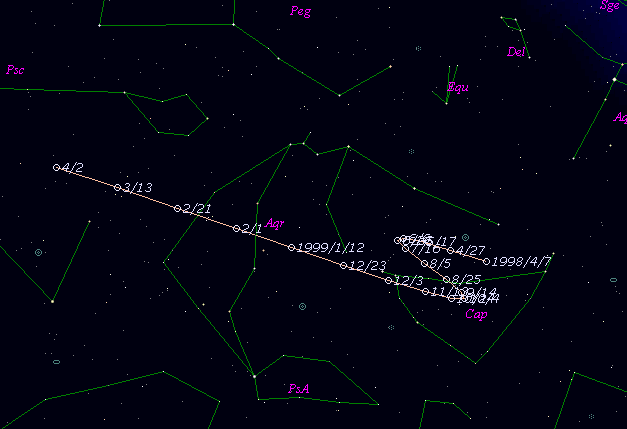# \$B%_%e%i!

136P/Mueller 3 (1999)###\$B%W%m%U%#!<%k(B

 \$BId9f(B 136P/1998 K4 \$B8!=PF|(B 1998\$BG/(B 5\$B7n(B24\$BF|(B \$B8!=P8wEY(B 20.6\$BEy(B \$B8!=P J. V. Scotti (Lunar and Planetary Laboratory)

###\$B50F;MWAG(B

```   The following improved orbital elements by Kenji Muraoka, are
from 82 observations 1991 to 1999, perturbations by 9 Plantes,
Moon and 5 minor planets were taken into account.
The mean residual is +/- 0.80 arc seconds.

Epoch  =  1999 Mar.  3.0  TT       JDT = 2451240.5
T  =  1999 Mar. 20.34453       +/- 0.00604 (m.e.) TT
Peri. =  225.41739                +/- 0.00129
Node  =  137.96645                +/- 0.00017   (2000.0)
Incl. =    9.41417                +/- 0.00003
q  =    3.0106147              +/- 0.0000011 AU
e  =    0.2886851              +/- 0.0000003
a  =    4.2324642              +/- 0.0000005 AU
n  =    0.11319158             +/- 0.00000002
P  =    8.707                  +/- 0.0000016  years
```

###\$B@1?^(B###\$B8wEYJQ2=(B

```        m1 = 0 + 5 log\$B&\$(B + 33 log r(t - 130)
```##### \$B50F;MWAG\$OB<2,7r<#;a\$N7W;;\$K\$h\$k\$b\$N\$G\$9!#(B \$B@1?^\$O(B StellaNavigator Ver.2.0 for Windows (\$B%"%9%H%m%"!<%D(B \$BJTCx(B / \$B%"%9%-!<=PHG6I4)(B) \$B\$G:n@.\$7\$?\$b\$N\$G\$9!#(B \$B8wEY%0%i%U\$O(BComet for Windows\$B\$G:n@.\$7\$?\$b\$N\$G\$9!#(B